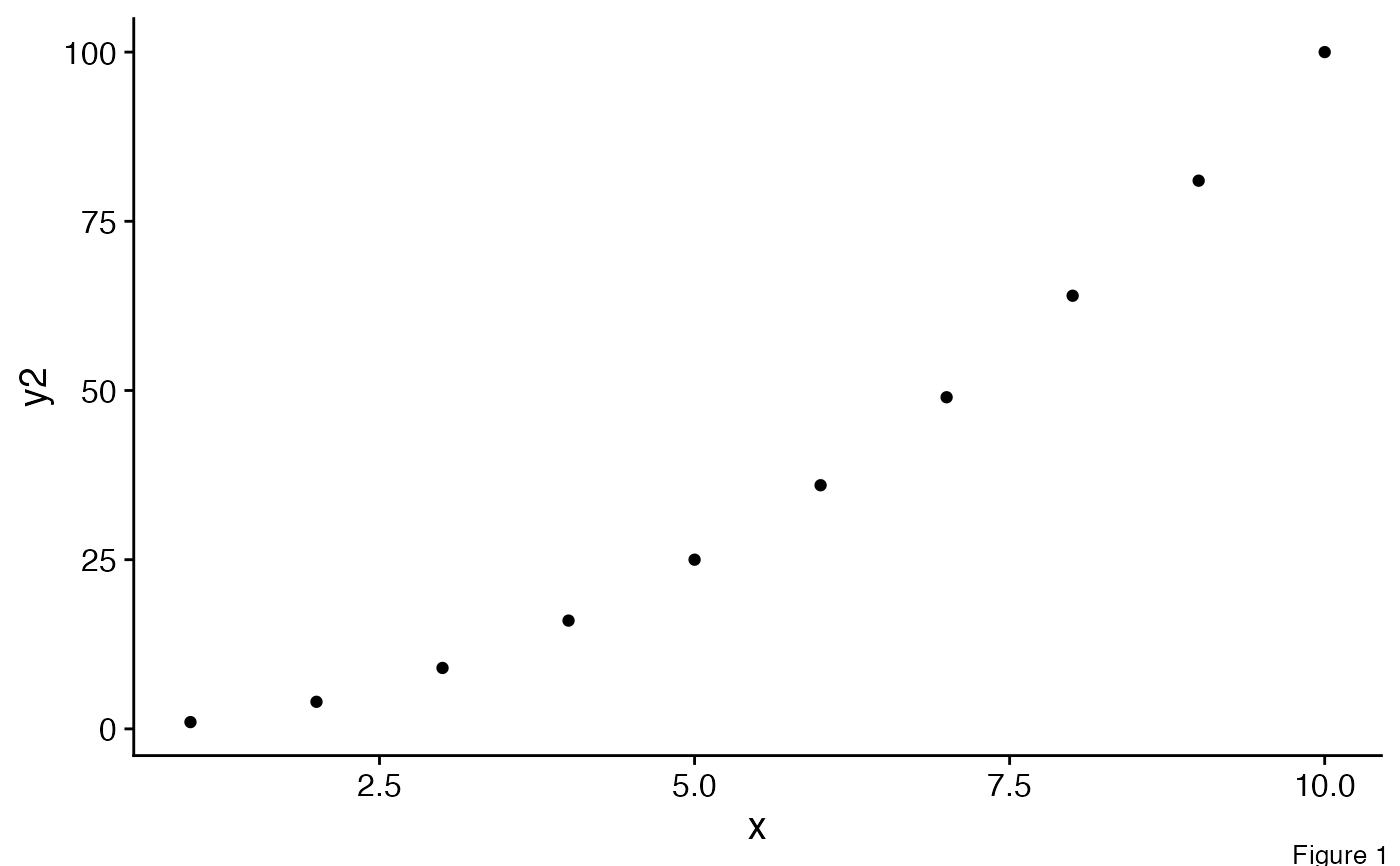The main purpose of this function is to add labels specifying extra information about the figure, such as "Figure 1", or "A" - often useful in cowplots with more than one pane. The function is similar to draw_plot_label.

draw_figure_label(label, position = c("top.left", "top", "top.right",
"bottom.left", "bottom", "bottom.right"), size, fontface, ...)

## Arguments

label Label to be drawn Position of the label, can be one of "top.left", "top", "top.right", "bottom.left", "bottom", "bottom.right". Default is "top.left" (optional) Size of the label to be drawn. Default is the text size of the current theme (optional) Font face of the label to be drawn. Default is the font face of the current theme other arguments passed to draw_plot_label

draw_plot_label

## Examples

library(ggplot2)
df <- data.frame(
x = 1:10, y1 = 1:10, y2 = (1:10)^2, y3 = (1:10)^3, y4 = (1:10)^4
)

p1 <- ggplot(df, aes(x, y1)) + geom_point()
p2 <- ggplot(df, aes(x, y2)) + geom_point()
p3 <- ggplot(df, aes(x, y3)) + geom_point()
p4 <- ggplot(df, aes(x, y4)) + geom_point()

# Create a simple grid
p <- plot_grid(p1, p2, p3, p4, align = 'hv')

# Default font size and position
p + draw_figure_label(label = "Figure 1")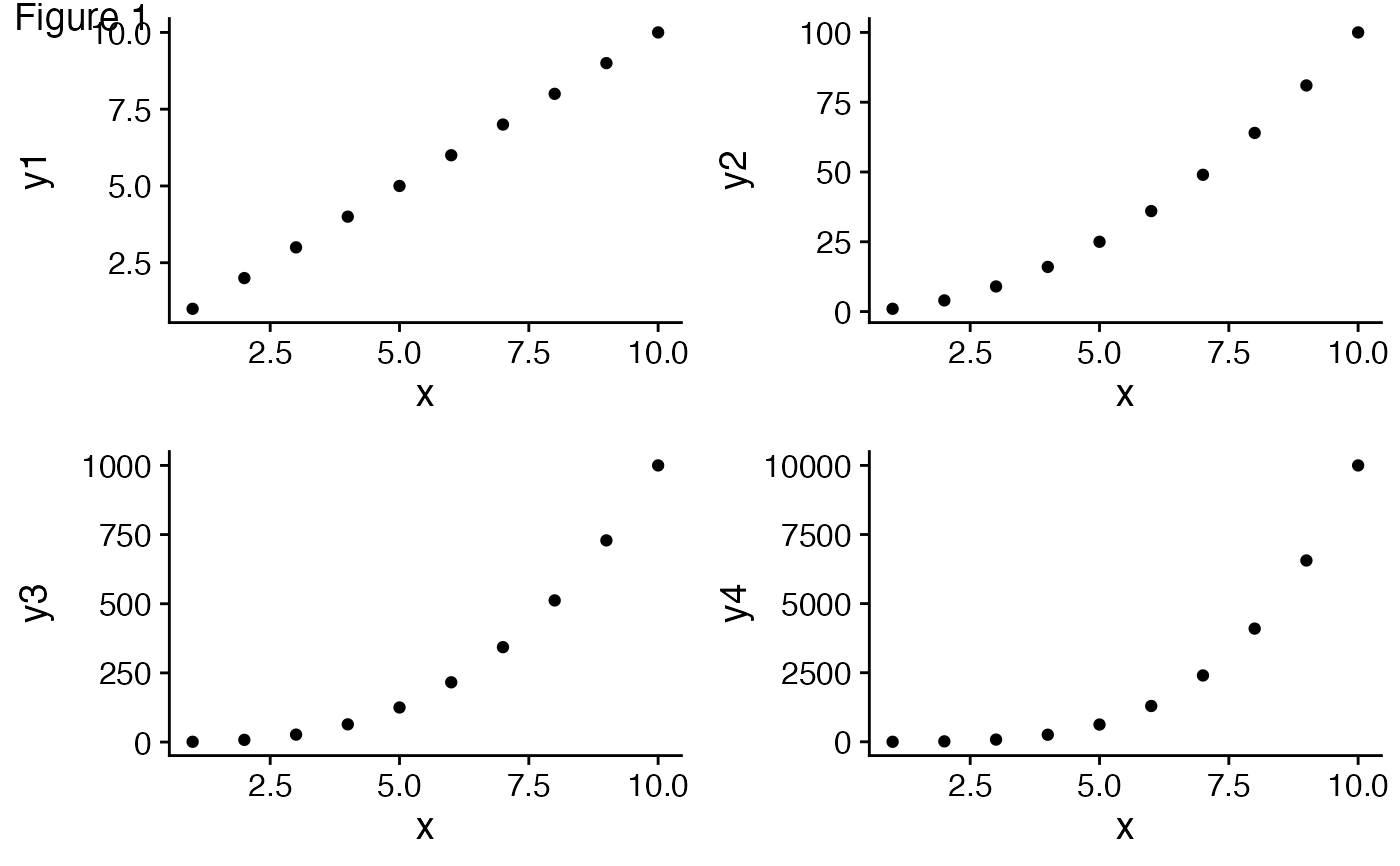# Different position and font size
p + draw_figure_label(label = "Figure 1", position = "bottom.right", size = 10)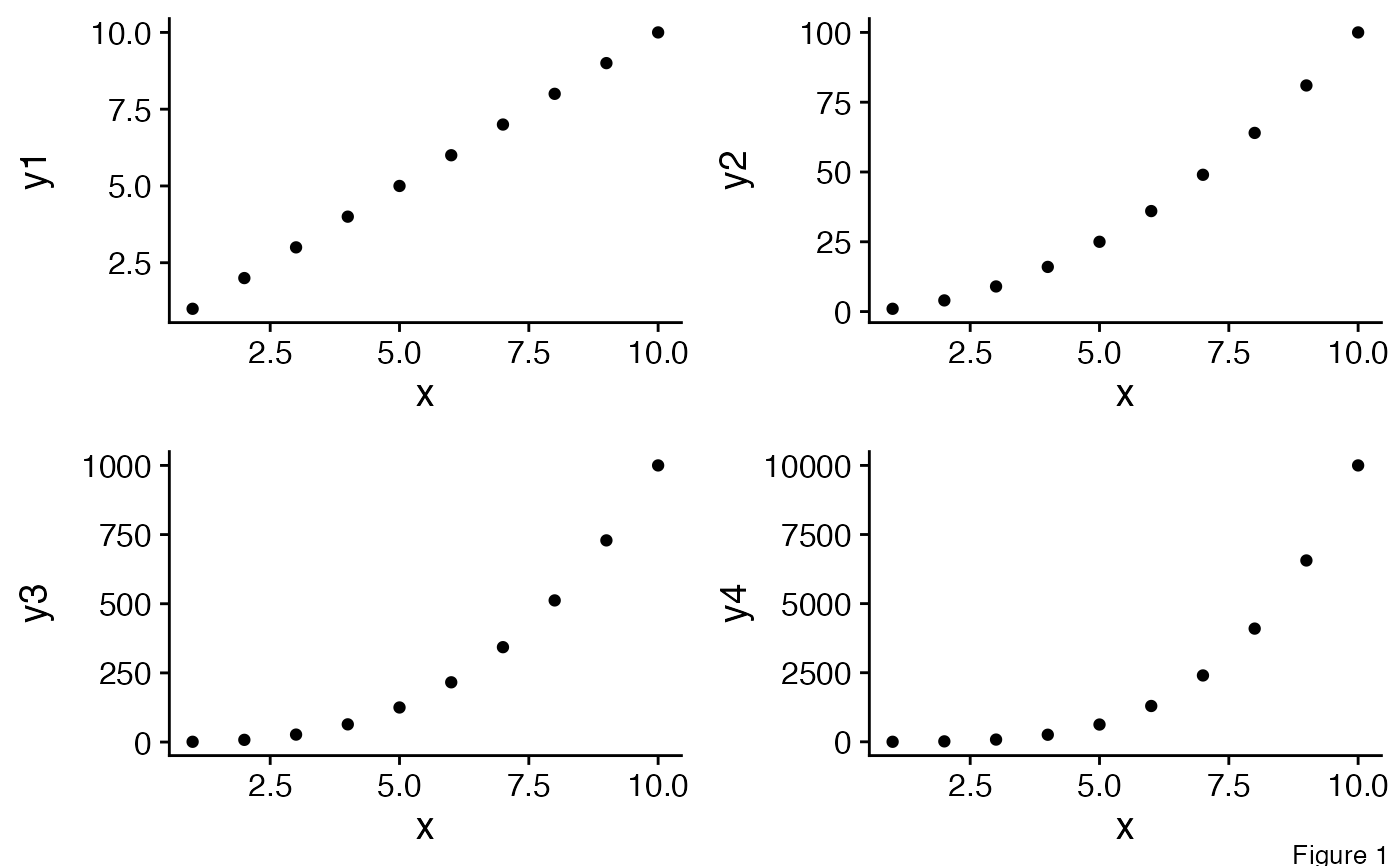# Using bold font face
p + draw_figure_label(label = "Figure 1", fontface = "bold")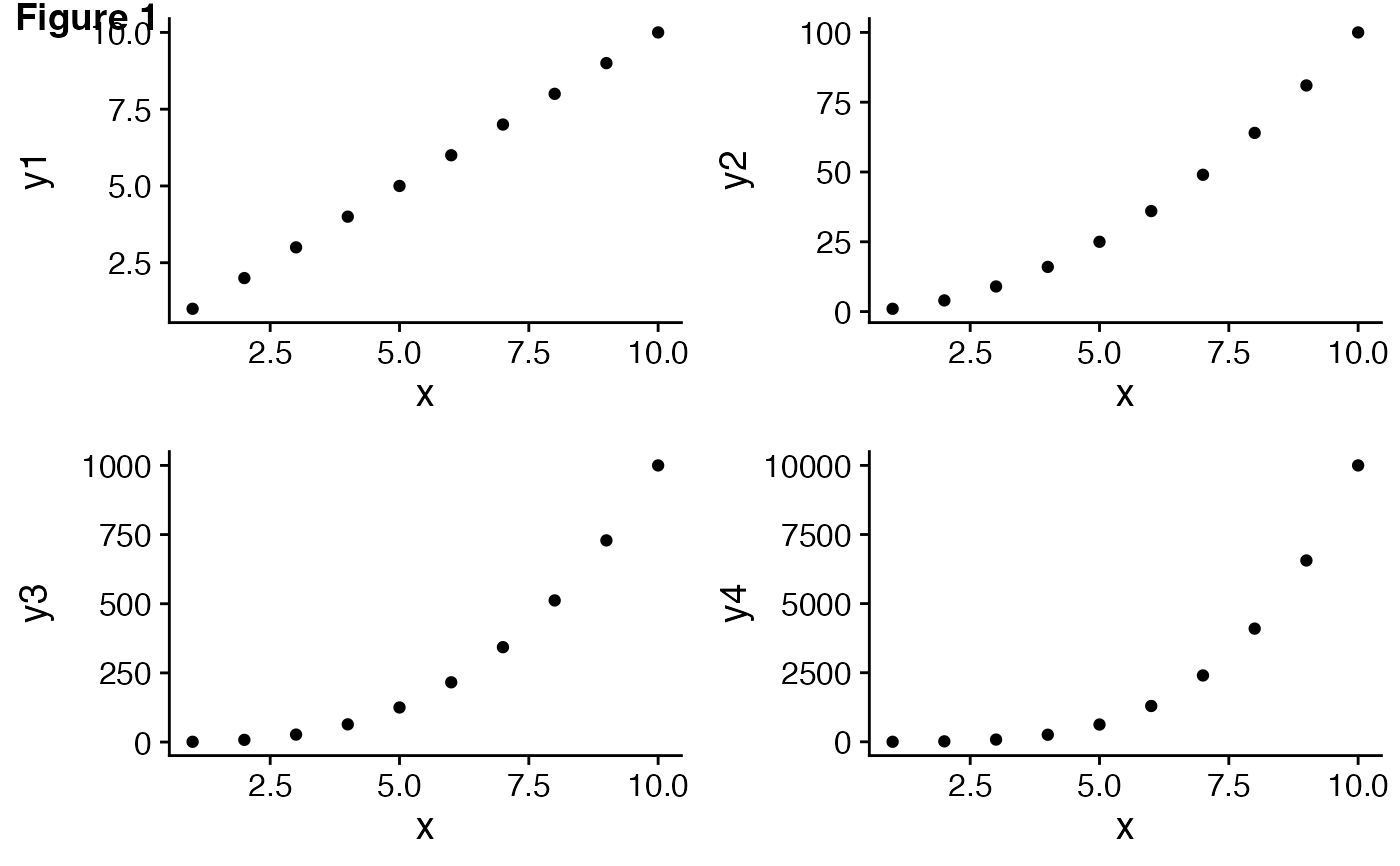# Making the label red and slanted
p + draw_figure_label(label = "Figure 1", angle = -45, colour = "red")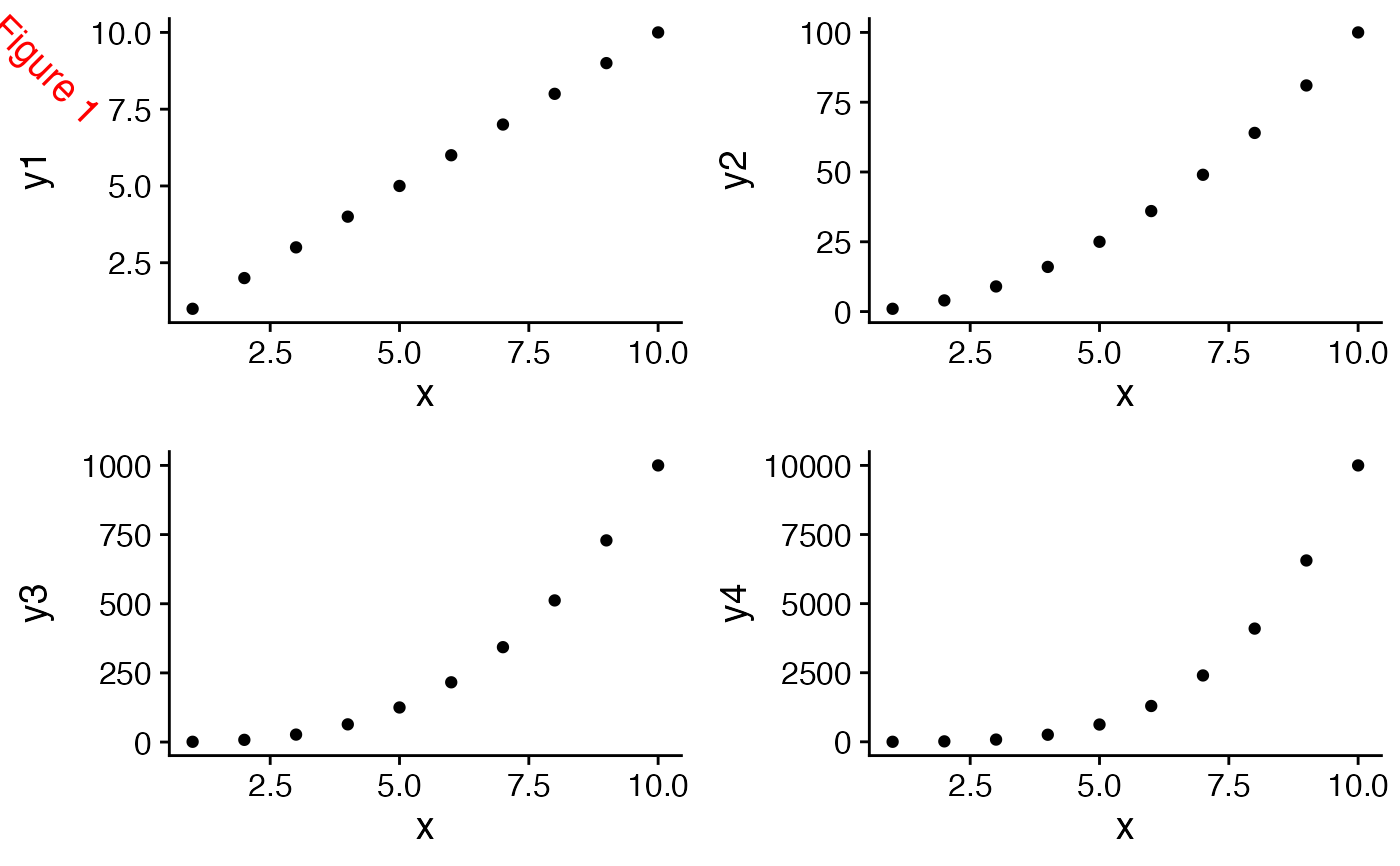# Labeling an individual plot
ggdraw(p2) + draw_figure_label(label = "Figure 1", position = "bottom.right", size = 10)